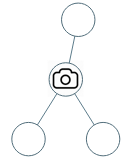# 968. Binary Tree Cameras

Given a binary tree, we install cameras on the nodes of the tree.

Each camera at a node can monitor its parent, itself, and its immediate children.

Calculate the minimum number of cameras needed to monitor all nodes of the tree.

Example 1:``````Input: [0,0,null,0,0]
Output: 1
Explanation: One camera is enough to monitor all nodes if placed as shown.
``````

Example 2:``````Input: [0,0,null,0,null,0,null,null,0]
Output: 2
Explanation: At least two cameras are needed to monitor all nodes of the tree. The above image shows one of the valid configurations of camera placement.
``````

Note:

1. The number of nodes in the given tree will be in the range `[1, 1000]`.
2. Every node has value 0.

``````class Solution {
public:
int minCameraCover(TreeNode* root) {
int res = 0;
return (helper(root, res) < 1 ? 1 : 0) + res;
}
// Return 0 if leaf, 1 if parent of leaf with camera on this node, 2 if covered without camera on this node.
int helper(TreeNode* node, int& res) {
if (!node) return 2;
int left = helper(node->left, res), right = helper(node->right, res);
if (left == 0 || right == 0) {
++res;
return 1;
}
return (left == 1 || right == 1) ? 2 : 0;
}
};
``````

Github 同步地址:

https://github.com/grandyang/leetcode/issues/968

Distribute Coins in Binary Tree

https://leetcode.com/problems/binary-tree-cameras/

https://leetcode.com/problems/binary-tree-cameras/discuss/211180/JavaC%2B%2BPython-Greedy-DFS

https://leetcode.com/problems/binary-tree-cameras/discuss/211966/Super-Clean-Java-solution-beat-100-DFS-O(n)-time-complexity

LeetCode All in One 题目讲解汇总(持续更新中…)

 微信打赏Venmo 打赏×

Help us with donation